• 线性回归模型，哪些变量取对数形式？
千次阅读
2020-12-23 13:14:01

并不是大的量和小的量放在一起大的就要对数，那我必然要问你，为什么不选择开N次方呢？或者把小的求阶乘(当然这种操作可能也不太“自然”)？

事实上选择如何处理特征是要有一定的“具象”理由的，常见的两个原因有：

一：在对变量和预测值做线性相关性分析以后发现不是线性关系。你可以简单的理解为，随便挑一个特征x_0，把y=f(x_0)画出图来一看，线是弯的，本来就不是直的。然后我们就可以根据数据来猜测甚至干脆试试到底是幂关系还是指数关系或是其他。尝试过以后再做线性相关性分析，看看是不是这么处理就线性了。

二：“量纲”统一，曾经我在做一个新词发现的操作时需要用到的两个指标分别是词频f和左/右邻字的分布熵e，如果从数学的角度上看我们显然不能把f+e当做一个指标，因为词频相当于是一种分布的概率值，而熵是概率的对数的期望。所以如果我们联想类比物理中“量纲”的概念，我们就可以很快想到，用log f+e可能会是一个可行的指标，至少他们的缩放是同级别的，都是概率的对数级别的。

最后，就是真的要理解业务。这个很重要。如果在工作中，就去和销售或客户代表聊聊，也许专业人士的经验会告诉你，在他们的领域中，早就有前人发现这些变量间大概的关系了。比如可能是个微分方程，其实很多现实规律都来源于一些差分方程，而差分方程常常能解出一堆带e的n次方的东西。

更多相关内容
• 包括指数、对数、幂指数、双曲线、Logistic、多项式、Gamma等模型的非线性拟合，每行代码均有中文注释便于理解
• 回归分析线性回归Logistic回归对数线性模型PPT学习教案.pptx
• SPSS专题回归分析线性回归Logistic回归对数线性模型PPT教案.pptx

BG：在box-cox变换中，当λ = 0时即为对数变换。

当所分析变量的标准差相对于均值而言比较大时，这种变换特别有用。对数据作对数变换常常起到降低数据波动性和减少不对称性的作用。。这一变换也能有效消除异方差性

library(MASS)

library(openxlsx)

attach(data)

op

plot(size,effort) #图4-4(a)

plot(log(size),log(effort)) #图4-4(b)

#绘制频率分布直方图

hist(effort) #图4-5(a)

hist(size) #图4-5(b)effor 和 size 这两个变量的频率分布图表明，它们并不满足正态分布。为了接近正态分布，必须变换这些变量(通过频率分布图判断变量是否满足正态分布)

1.先进行基本的线性回归，利用得到的模型进行box-cox变换

lm1=lm(effort~size+t14) #拟合线性回归模型

summary(lm1)

#绘制残差图进行残差分析

plot(fitted(lm1),resid(lm1),cex=1.2,pch=21,col="red",bg="orange",xlab="Fitted value",ylab="Residuals")

boxcox(lm1,lambda=seq(0,1,by=0.1)) #进行box-cox变换从残差图可以看到误差项不满足Gauss-Markov假设。

右图的Box-Cox变换建议问哦们λ可以取在[0.05,0.6]范围内，对投入工作量(effort) 取对数有一定的可信度(λ=0 几乎落在置信域内)

进行对数变换

lm2=lm(log(effort)~size+t14)

summary(lm2)

#绘制残差图

plot(fitted(lm2),resid(lm2),cex=1.2,pch=21,col="red",bg="orange",xlab="Fitted value",ylab="Residuals")书上的结果时残差范围大致在[-25,40]内，不满足Gauss-Markov假设

与书上结果不符，上图参擦汗图表示这个模型是可行的。

2.试图拟合 effort 与 log(size)，t14 的回归方程。

lm3=lm(effort~log(size)+t14)

summary(lm3)

#绘制残差图

plot(fitted(lm3),resid(lm3),cex=1.2,pch=21,col="red",bg="orange",xlab="Fitted value",ylab="Residuals")

#box-cox变换求λ

boxcox(lm3,lambda=seq(0,1,by=0.1))根据右图，Box-Cox变换建议我们取 λ=0

建立如下方程 ln(effort) = β0 + β1ln(size) + β2 t14 + e

lm4=lm(log(effort)~log(size)+t14) #进行线性回归

summary(lm4)

#绘制残差图

plot(fitted(lm4),resid(lm4),cex=1.2,pch=21,col="red",bg="orange",xlab="Fitted value",ylab="Residuals")

#进行box-cox变换

boxcox(lm4,lambda=seq(0,1,by=0.1))因为λ=1 包含在box-cox图像所示的置信域内，说明不进行变换也是ok的

而且通过残差分析，可以看出这个模型是合理的。

展开全文• 常用的三个线性模型的原理及python实现——线性回归（Linear Regression）、对数几率回归（Logostic Regression）、线性判别分析（Linear Discriminant）。 这可能会是对线性模型介绍最全面的博客一、线性模型 ...

常用的三个线性模型的原理及python实现——线性回归（Linear Regression）、对数几率回归（Logostic Regression）、线性判别分析（Linear Discriminant）。 这可能会是对线性模型介绍最全面的博客

## 一、线性模型 （Linear Model）

对于一个具有d个属性的对象来说，我们可以用下面这一组向量描述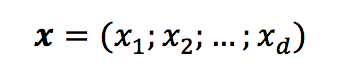向量中的每个值都是对象一个属性的值，由上可以看出，线性模型是一个通过属性的线性组合来进行对象判定的函数，即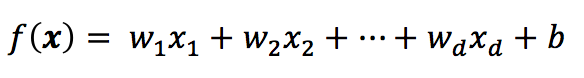用向量的形式可以写成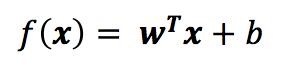其中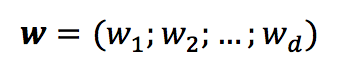当求得wb后，线性模型就得以确定。线性模型是最简单也是最常用的模型之一，同时，很多更为强大的非线性模型也是由线性模型发展来的，所以，了解掌握线性模型十分必要

## 二、线性回归 （Linear Regression）

线性回归是指，模型试图学得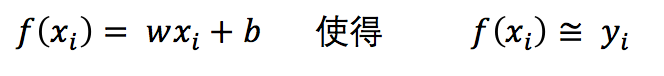求解模型的关键在于，如何求得wb，解决问题的落点在于，如何衡量f(x)y 差距，通常我们使用均方误差来衡量这种差距的大小，即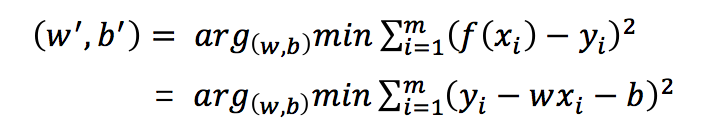均方差有非常良好的几何意义，它对应了常用的欧几里得距离即“欧式距离”。基于均方误差最小化的原理来求解模型的方法叫做“最小二乘法”（least square method），在线性回归中，最小二乘法就是试图找到一条直线，使所有样本到直线上的欧式距离之和最小，现令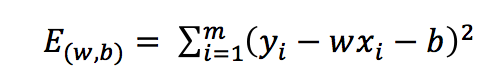将其对wb分别求导，并令其导数为0，可得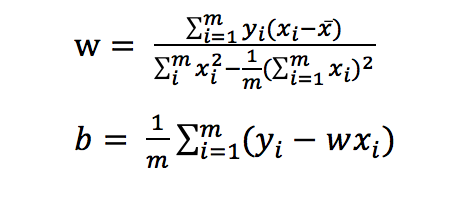对于更一般的情形，若样本由d个属性描述，此时我们应当求解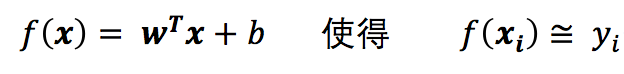这就是我们通常所说的多元线性回归（multivariate linear regression）
现在利用最小二乘法对w和b进行估计。将wb统一记为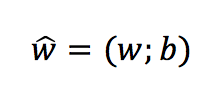同时也把数据集D表示为一个 *m x (d + 1)*的矩阵 X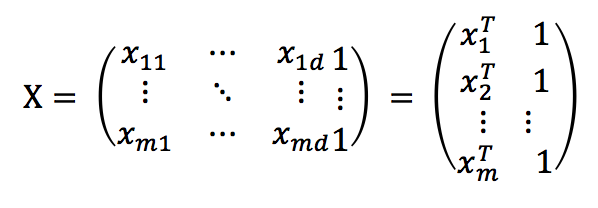将标记也写成向量形式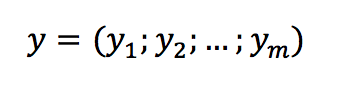此时的求解方程可以写为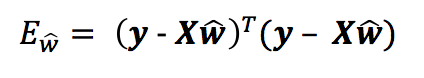用上式对w进行求导，当X 的转置与其相乘为满秩矩阵或者正定矩阵时，令导数为0可得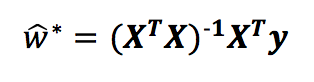最终得到的多元线性回归模型如下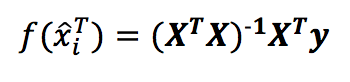基于上述理论，其python源码如下：


'''
1.using least square method figure out linear regression
'''
import numpy as np
import matplotlib.pyplot as plt

def linear_regression(x,y):
def preprocess(x):
X = np.mat(x)
b = np.mat(*len(x))
X = np.hstack((X,b.T))
return X

def cal_w(x,y):
X = preprocess(x)
Y = np.mat(y).T
return (X.T*X).I*X.T*Y

return preprocess(x)*cal_w(x,y),cal_w(x,y).tolist()


其测试代码如下（注本文所有的代码原为一个文件，运行时，请完整复制全部代码）


#visiable and output test
x = [[0.697,0.460],[0.774,0.376],[0.634,0.264],[0.608,0.318],[0.556,0.215],[0.403,0.237],[0.481,0.149],
[0.437,0.211],[0.666,0.091],[0.243,0.267],[0.245,0.057],[0.343,0.099],[0.639,0.161],[0.657,0.198],
[0.360,0.370],[0.593,0.042],[0.719,0.103]]
y = [1,1,1,1,1,1,1,1,0,0,0,0,0,0,0,0,0]
yy,w = linear_regression(x,y)
i,x1,x2 = 0,[],[]
while i < len(x):
x1.append(x[i])
x2.append(x[i])
i += 1

i = 0
plt.figure(1)
plt.subplot(121)
plt.title('linear regression')
while i < len(x1):
if y[i] == 0:
plt.scatter(x1[i],x2[i],color = 'r')
elif y[i] == 1:
plt.scatter(x1[i],x2[i],color = 'g')
i += 1
a = -(w/w)
b = -(w+w)/w
plt.plot([0,1],[a,b])


## 三、对数几率回归（Logistic Regression）

对数几率回归实质上使用Sigmoid函数对线性回归的结果进行了一次非线形的转换，Sigmoid函数如下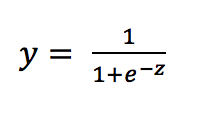将线性模型带入可得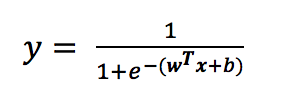该式实际上是在用线性回归模型的预测结果去逼近真实标记的对数几率，因此，其对应的模型称为“对数几率回归”（logistic regression），将上式取常用对数，可转化为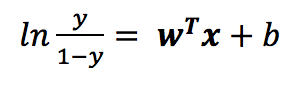现在我们开始对wb进行估计，将上式中的y视为类后验概率估计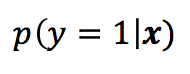则可以将模型转化为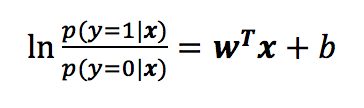显然有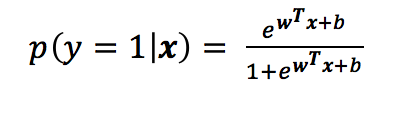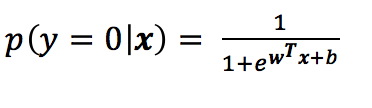到这里，我们便可以使用“极大似然法”（maximum likelihood method）来估计wb，给定数据集如下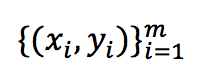对率回归模型最大化“对数似然”,记为式 1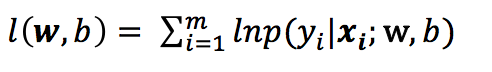即令每个样本属于其真实标记的概率越大越好，现令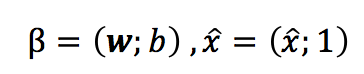再令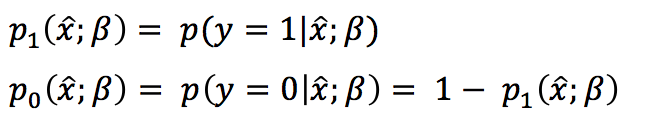此时，便可将之前式1里的似然项改写为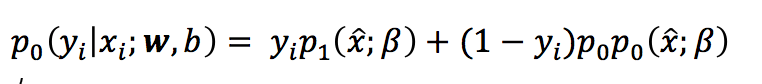由此可知，式1等于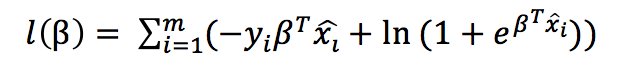这是一个高阶可导连续凸函数，接下来给出牛顿法的推导公式，首先为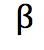设置任意值
设定允许误差，再代入下式循环求解求解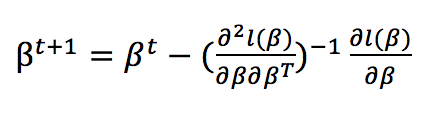其中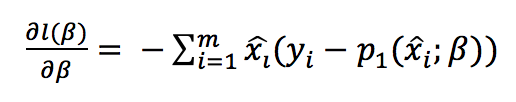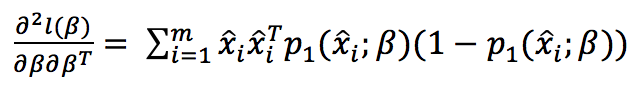基于上述理论，其python源码如下：

'''
2.Newton method figure out logistic regression
'''

def logistic_regression(x,y,error,n):
def preprocess(x,y):
X = np.mat(x)
b = np.mat(*len(x))
X = np.hstack((X,b.T))
w = *(len(x)+1)
W = np.mat(w).T
Y = y
return X,W,Y

def func_p(X,W):
a = (X*W).tolist()
b = float(a)
temp = np.exp(b)/(1+np.exp(b))
return temp

def dfunc(X,Y,W):
i,num,sum1 = 0,len(X),0
while i < num:
temp = Y[i] - func_p(X[i],W)
sum1 += X[i]*temp
i += 1
return sum1*(-1)

def d2func(X,Y,W):
i,num,sum1 = 0,len(X),0
while i < num:
temp = func_p(X[i],W)*(1 - func_p(X[i],W))
sum1 += X[i]*(X[i].T)*temp
i += 1
sum1 = sum1.tolist()
return float(sum1)

def Newton(x,y,error,n):
X,W,Y = preprocess(x,y)
i = 1
while i < n:
d1 = dfunc(X,Y,W)
a = (d1*d1.T).tolist()
a = float(a)
if a < error:
return W
break
temp = dfunc(X,Y,W)
W = W - temp.T*(d2func(X,Y,W)**(-1))
i += 1
if i == n:
return 'error'

w = Newton(x,y,error,n)
X,W,Y = preprocess(x,y)
yy = (X*w).tolist()
w = w.tolist()
return w,yy



测试代码如下


#visiable and output test
w,yy = logistic_regression(x,y,0.0001,1000)

i,x1,x2,z = 0,[],[],[]
while i < len(x):
x1.append(x[i])
x2.append(x[i])
z.append(yy[i])
i += 1

i = 0

plt.subplot(122)
plt.title('logistic regression')
while i < len(x1):
if y[i] == 0:
plt.scatter(x1[i],x2[i],color = 'r')
elif y[i] == 1:
plt.scatter(x1[i],x2[i],color = 'g')
i += 1
a = -(w/w)
b = -(w+w)/w
plt.plot([0,1],[a,b])


## 四、线性判别分析（Linear Discriminant Analysis）

线性判别分析是一种经典的线形学习方法，最早由费舍尔（Fisher）在二分类问题上提出，所以也称为“Fisher判别分析”
其思想非常朴素：给定训练样例集，设法将样例点投影到一条直线上，使得同类样例的投影点尽可能接近、异类样例的投影点尽可能远离；在对测试样例进行分类时，将其投影到同样的这条直线上，再根据投影点的位置来确定新样本的类别。这里只讲述二分类问题的原理和求解，给定数据集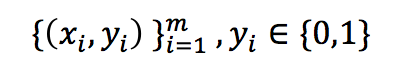再分别令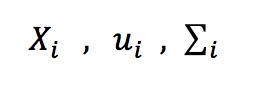表示i（等于0或1）类示例的集合、均值向量、协方差矩阵，将数据投影到直线w上，则两类样本的中心在直线上的投影分别为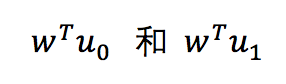若将所有样本点都投影到直线上，则两类样本的协方差分别为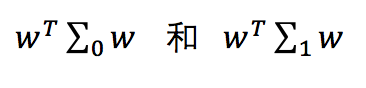想要使同类样例的投影点尽可能接近，可以让同类样例的协方差尽可能小，即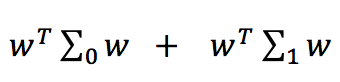尽可能小；同时，也想要让异类样例的投影点尽可能远离，可以让类中心之间的距离尽可能大，即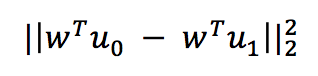尽可能的大，则可以定义最大化目标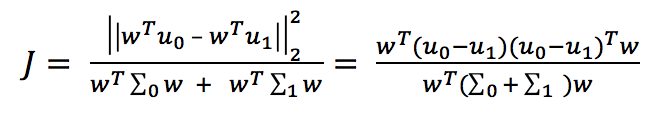定义类内散度矩阵（within class scatter matrix）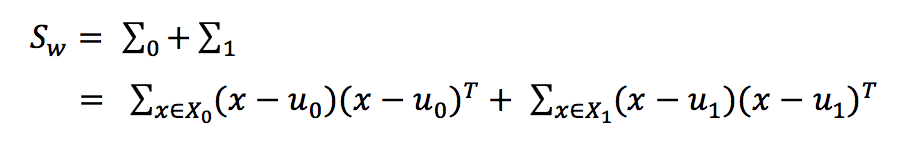以及类间散度矩阵（between class）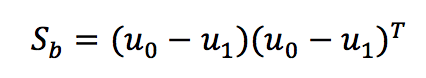可将最大化目标重写为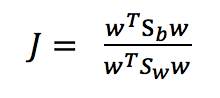由拉格朗日乘法子可从上式求出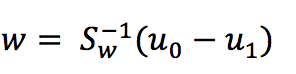由此可解出二分类问题的LDA模型，基于上述理论，其python源码如下：

'''
3.Linear Discriminant Analysis for binary classification problem
'''

def LDA(x,y):
def preprocess(x,y):
i = 0
X0,X1 = [],[]
while i < len(y):
if y[i] == 0:
X0.append(x[i])
elif y[i] == 1:
X1.append(x[i])
i += 1
return X0,X1

def average(X):
X = np.mat(X)
i = 1
while i < len(X):
X = X + X[i]
i += 1
res = X/i
return res

def Sw(X0,X1,u0,u1):
X_0 = np.mat(X0)
X_1 = np.mat(X1)
Sw0,i = 0,0
temp0 = (X_0 - u0).T*((X_0 - u0))
#        while i < len(temp0):
#            Sw0 += float(temp0[i,i])
#            i += 1
#        Sw1,i = 0,0
temp1 = (X_1 - u1).T*((X_1 - u1))
#        while i < len(temp1):
#            Sw1 += float(temp1[i,i])
#            i += 1
#        return Sw0+Sw1
return temp0 + temp1

X0,X1 = preprocess(x,y)
u0,u1 = average(X0),average(X1)

SW = Sw(X0,X1,u0,u1)
return (SW**(-1)*numpy.mat(u0-u1).T).tolist()

#visiable and output test
W = LDA(x,y)

i,x1,x2,z = 0,[],[],[]
while i < len(x):
x1.append(x[i])
x2.append(x[i])
i += 1
i = 0
plt.figure(2)
plt.subplot(121)
plt.title('LDA')
while i < len(x1):
if y[i] == 0:
plt.scatter(x1[i],x2[i],color = 'r')
elif y[i] == 1:
plt.scatter(x1[i],x2[i],color = 'g')
i += 1
print(W)

plt.plot([0,-W],[0,-W])


## 五、三个模型结果对比图

完整代码地址：https://github.com/LiangjunFeng/Machine-Learning/blob/master/1.linear_model.py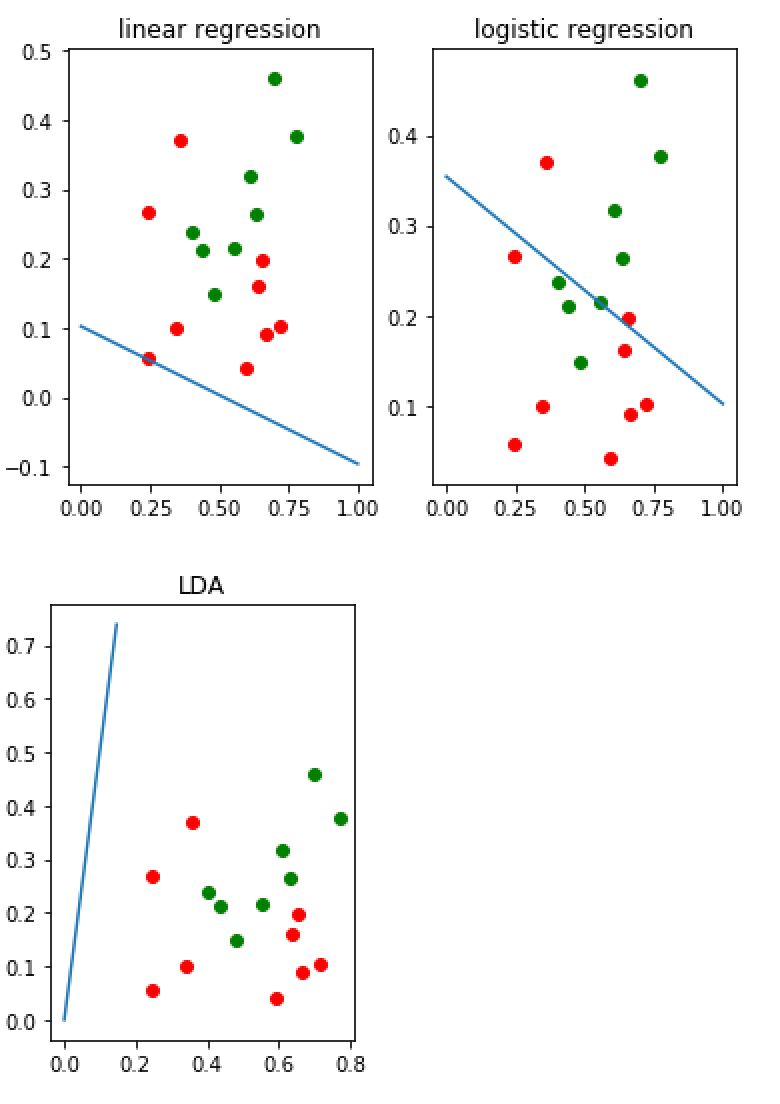## 六、更多资源下载

微信搜索“老和山算法指南”获取更多下载链接与技术交流群有问题可以私信博主，点赞关注的一般都会回复，一起努力，谢谢支持。

展开全文python lda算法原理
• 对数线性回归； y的衍生物 3.1 基本形式 假设样本x有d个属性，线性模型（linear model）试图学得一个通过属性的线性组合来进行预测的函数，即f(x)=w1x1+w2x2+⋅⋯+wdxd+bf(x)=w1x1+w2x2+⋅⋯+wdxd+bf(x)=w_{1}x_...

墙裂推荐阅读：ｙ的衍生物

关键词：最小二乘法；正则化；对数线性回归； y的衍生物

## 3.1 基本形式

假设样本x有d个属性，线性模型（linear model）试图学得一个通过属性的线性组合来进行预测的函数，即 f(x)=w1x1+w2x2++wdxd+b f ( x ) = w 1 x 1 + w 2 x 2 + ⋅ ⋯ + w d x d + b $f(x)=w_{1}x_{1} + w_{2}x_{2}+\cdot \cdots +w_{d}x_{d}+b$ ，向量形式 f(x)=wTx+b f ( x ) = w T x + b $f(x)=w^{T}x+b$

## 3.2 线性回归

关键词：无序属性连续化。
对离散属性，若属性值之间存在“序”（order）关系，可通过连续化将其转化为连续值，例如二值属性身高的取值，“高”“矮”可和转化为｛1.0 ， 0｝。 若属性值之间不存在序的关系，例如属性“瓜类”的取值为西瓜，南瓜，冬瓜，则可转化为（0，0，1），（0，1，0），（1，0，0）。

关键词：最小二乘法（least square method）。
基于均方误差最小化来进行模型求解的方法称为“最小二乘法”。在线性回归中，最小二乘法就是试图找到一条直线，使所有样本到直线上的欧氏距离之和最小。

关键词： 正则化（regularization）项。
假设解一个线性方程组，当方程数大于自由变量数时，是没有解的。反过来，当方程数小于自由变量数的时候，解就有很多个了。往往，我们会碰到这种情况，参数多，“方程”少的情况，那么有很多个w（权值向量）都能使均方误差最小，那么该选哪一个呢？ 这就涉及到 归纳偏好问题了，常见的做法是引入正则化项。

关键词：对数线性回归（log-linear regression）；y的衍生物
把线性回归模型简写为： f(x)=wTx+b f ( x ) = w T x + b $f(x)=w^{T}x+b$ ，当我们希望线性模型的预测值逼近真实标记y，这样就是线性模型。那可否令模型的预测值毕竟y的衍生物呢？ 作者的这一描述实在太妙了！y的衍生物，通俗易懂！ 假设y的衍生物是 y的对数即lny，那么就可以得到对数线性回归模型： lny=wTx+b l n y = w T x + b $lny=w^{T}x+b$ ， 也就是让模型 去逼近 lny，而不是y。也可以对 lny=wTx+b l n y = w T x + b $lny=w^{T}x+b$ 做一下变换就变成了 y=ewTx+b y = e w T x + b $y=e^{w^{T}x+b}$，也可以理解为让 ewTx+b e w T x + b $e^{w^{T}x+b}$ 去逼近y。形式上还是线性回归的，但实质上已是在求取输入空间到输出空间的非线性函数映射。如图：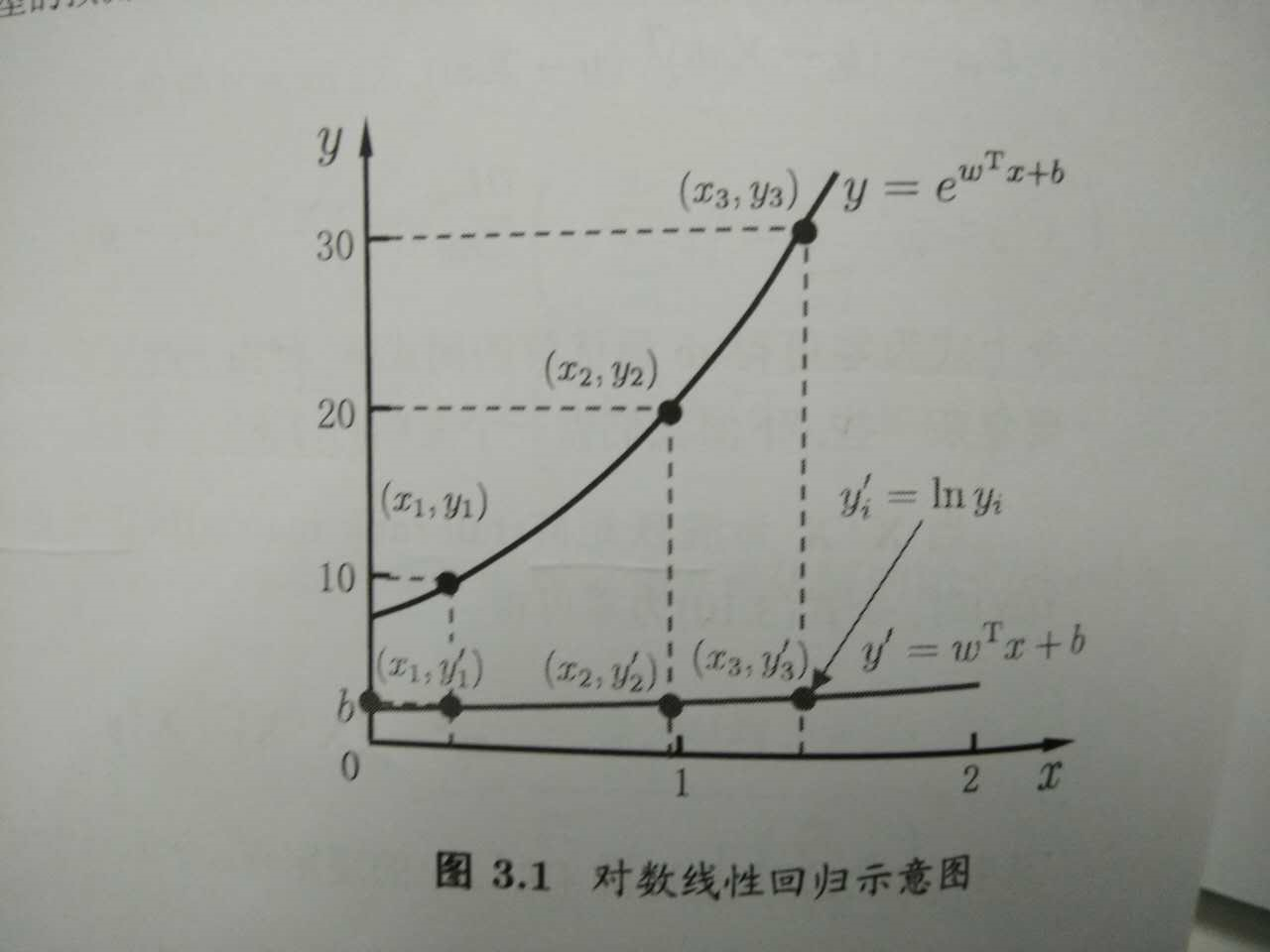来思考一个问题
想从线性模型出发，去扩展线性模型，就是让线性模型 f(x)=wTx+b f ( x ) = w T x + b $f(x)=w^{T}x+b$去拟合y的衍生物，那么我们常说的逻辑回归（对数几率回归）是怎么从线性模型演变而来的呢？是让 wTx+b w T x + b $w^{T}x+b$去拟合哪一种“ｙ的衍生物” 什么呢？这个可以思考思考后，请看下篇：逻辑回归

展开全文机器学习
• 如果是分类任务，如何使用线性回归模型呢？答案在广义线性模型的公式中，只需要找到一个单调可微函数将分类任务的真实标记y 与线性回归模型的预测值联系起来。 考虑二分类任务，输出 $y \in${0,1} , 线性回归的预测...
• 二项Logistic回归模型由条件概率分布P(Y|X)表示，X为随机变量，取值为实数，Y同为随机变量，但取值为1或0； 二项 Logistic回归模型的条件概率分布： 其中，w称为权值向量，b为偏置，x为输入，Y为输出...
• 每当我们估计回归模型时，都必须假定回归具有正确的函数形式。该假设可能会有以下几种错误：■可以从回归中忽略一个或多个重要变量。■在估计回归之前，可能需要转换一个或多个回归变量(例如，通过对变量取自然对数)...
• 简单线性回归模型 打开Eviews软件，可以选择建立一个new workfile，也可以选择打开一个已存在的workfile。 选择数据类型： Unstructured/Undated 横截面数据 Dated-regular freguency 时间序列数据（有固定...回归 算法
• 对数几率回归（logistic regression），有时候会译为逻辑回归(音译)，其实是我们把回归模型应用到分类问题时，线性回归的一种变形，主要是针对二分类提出的。既然是线性回归的一种变形，那么在理解对数几率回归时，...
• 以航运市场中重要船型-好望角型散货船为研究对象，在分析相关可量化因素与新船价格关系的基础之上，构建双对数线性回归模型分析各因素对新船船价的影响程度，发现对于新造好望角型散货船而言，二手船船价、新造船...
• 线性回归回归分析实质上就是研究一个或多个自变量X对一个因变量Y(定量数据)的影响关系情况。当自变量为1个时，是一元线性回归，又称作简单线性回归；自变量为2个及以上时，称为多元线性回归。在SPSSAU里均是使用...
• 常见的广义线性模型有：probit模型、poisson模型对数线性模型等等。对数线性模型里有：logistic regression、Maxinum entropy。本篇是对逻辑回归理论和应用的学习总结、以及广义线性模型导出逻辑回归的过程，下一...
• sklearn:线性回归与逻辑回归（对数几率回归）sklearn 机器学习
• 对数线性模型看到上面的交叉单元格，以及单元格内的频数数据，你是否很快就会联想到可以使用卡方检验来分析分类变量A和分类变量B的相关关系？上面这个表只有一个行变量和一个列变量，因此使用卡方检验非常方便快捷，...
• 机器学习专题(一)梯度下降法实现对数几率回归模型优化 文章目录机器学习专题(一)梯度下降法实现对数几率回归模型优化一、梯度下降算法二、使用步骤1.引入库2.读入数据总结 一、梯度下降算法 示例：pandas 是基于...机器学习 深度学习
• 下面是一个教程，介绍如何在Excel中构建线性回归模型以及如何解释结果 介绍 Excel真的能构建预测模型？ 这通常是我提起这个话题时的第一反应。当我演示如何利用Excel的灵活性为我们的数据科学和分析项目构建预测...
• 《机器学习：公式推导与代码实践》鲁伟著读书笔记。...在对数几率回归中，我们需要将线性回归模型的预测值转化为0/1值，而不是去逼近真实标签yyy。而取值范围为（0，1），单调可微的Sigmoid函数便是对机器学习 python 分类算法
• 线性回归（linear regression：试图学得一个线性模型以尽可能准确地预测实值输出标记。 1.最简单的形式：输入属性的数且只有一个， 最小二乘法：基于均方差误差最小化来进行模型的求解，在线性回归中，最小二乘法...机器学习 深度学习
• @机器学习之 线性回归对数几率回归（逻辑回归），线性判别分析 属性特征处理： 二值离散特征（0和1） 有序连续特征（1，2，3对应小 中 大） 无序离散特征（one-hot编码） 线性回归 均方误差最小化（最小二乘...机器学习
• 本文介绍对数几率回归模型，是一个典型的二分类任务学习模型 书接上回，我们可以对一个数据集得到它的回归方程 我们是这样去解读回归方程的：在相关属性下对样例的某一特征的度量 我们根据回归方程得到的特征度量...机器学习 梯度下降法
• 一般线性模型和线性回归模型Interpretability is one of the biggest challenges in machine learning. A model has more interpretability than another one if its decisions are easier for a human to ...机器学习 python 人工智能 深度学习
• 回归模型包含一个因变量和一个自变量时，我们称为简单线性回归 当只有一个预测变量，但同时包含变量的幂（比如，X、X 2、X 3）时，我们称之为多项式回归 当有不止一个预测变量时，则称为多元线回归 数据挖掘
• 他们展示了对数距离无线电传播模型的非线性回归分析（最小二乘优化）。 您需要实际的测量来运行它。 以CSV格式提供了一组大约1000个根据经验收集的信号强度测量值。 您只需要在 MATLAB 和 Python 中运行“log_...matlab
• 线性回归公式推导线性模型线性回归一元线性回归公式推导多元线性回归公式推导对数几率回归介绍对数几率回归公式推导 线性模型 线性回归 一元线性回归公式推导 多元线性回归公式推导 对数几率回归 介绍 对数几率回归...机器学习
• 一、线性回归的理论1)线性回归的基本概念线性回归是一种有监督的学习算法，它介绍的自变量的和因变量的之间的线性的...线性回归模型如下：对于线性回归的模型假定如下：(1) 误差项的均值为0，且误差项与解释变量之间...
• 包括指数、对数、幂指数、双曲线、Logistic、多项式、Gamma等模型的非线性拟合，每行代码均有中文注释便于理解
• 用R语言进行数据分析：常规和广义线性模型... fm2 将会拟合 y 对 x1 和 x2 的多重回归模型(和一个隐式的截距项)。一个重要的(技术上可选)参数是data = production。它指定任何构建这个模型的参数首先必须来自数据框......